# Precalculus : Convert Rectangular Coordinates To Polar Coordinates and vice versa

## Example Questions

1 3 Next →

### Example Question #75 : Polar Coordinates

Which polar-coordinate point is not the same as the rectangular point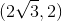?

Possible Answers: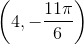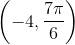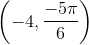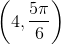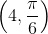Correct answer:Explanation:

Plotting this point creates a triangle in quadrant I: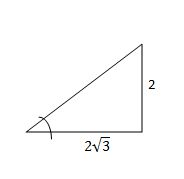Using our knowledge of Special Right Triangles, we can conclude that the angle is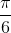and the radius/hypotenuse of this triangle is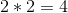. Our polar coordinates are therefore, so we can eliminate that as a choice since we know it works.

Looking at the unit circle [or just the relevant parts] can give us a sense of what happens when the angles and/or the radii are negative: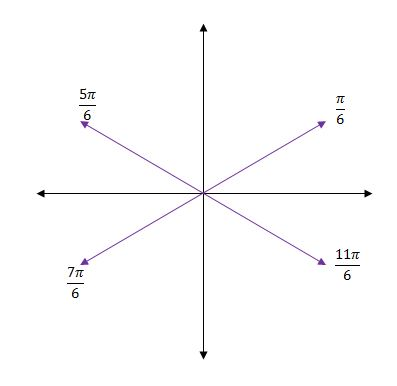Now we can easily see that the angle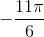would correspond with our angle of, soworks.

We can see that if our radius is negative we'd want to start off at the angle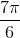, so the pointworks.

As we can see from looking at this excerpt from the unit circle, another way of writing the anglewould be to write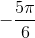, so the point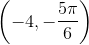works.

The only one that does not work would bebecause that would place us in quadrant II rather than I like we want.

### Example Question #76 : Polar Coordinates

Which of the following is a set of polar coordinates for the point with the rectangular coordinates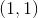Possible Answers: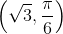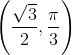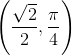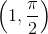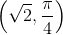Correct answer:Explanation:

The relation between polar coordinates and rectangular coordinates is given by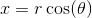and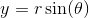.

You can plug in each of the choices forand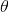and see which pair gives the rectangular coordinate.

The answer turns out to be.

Alternatively, you can findby the equation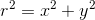, thus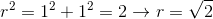.

As for finding, you can use the equation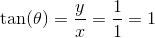, and since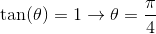.

Thus, the polar coordinate is### Example Question #77 : Polar Coordinates

Convert the point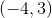to polar form

Possible Answers: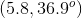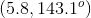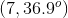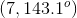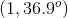Correct answer:Explanation:

First, find r using pythagorean theorem,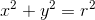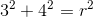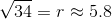Then we can find theta by doing the inverse tangent of y over x: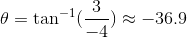Since this point is in quadrant II, add 180 degrees to get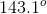### Example Question #78 : Polar Coordinates

Convert the following rectangular coordinates to polar coordinates: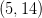Possible Answers: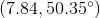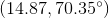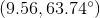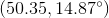Correct answer:Explanation:

To convert from rectangular coordinates to polar coordinates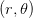: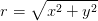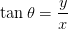Using the rectangular coordinates given by the question,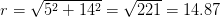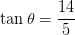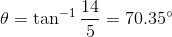The polar coordinates are1 3 Next →

### All Precalculus Resources